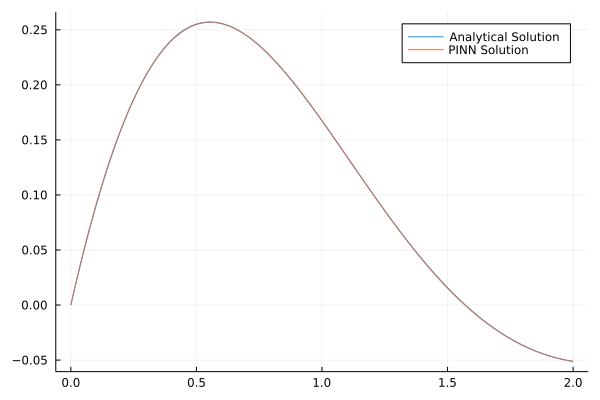# Solving Integro Differential Equations with Physics-Informed Neural Networks (PINNs)

The integral of function u(x),

$$$\int_{0}^{t}u(x)dx$$$

where x is variable of integral and t is variable of integro differential equation,

is defined as

using ModelingToolkit
@parameters t
@variables i(..)
Ii = Symbolics.Integral(t in DomainSets.ClosedInterval(0, t))

In multidimensional case,

Ix = Integral((x,y) in DomainSets.UnitSquare())

The UnitSquare domain ranges both x and y from 0 to 1. Similarly a rectangular or cuboidal domain can be defined using ProductDomain of ClosedIntervals.

Ix = Integral((x,y) in DomainSets.ProductDomain(ClosedInterval(0 ,1), ClosedInterval(0 ,x)))

## 1-dimensional example

Lets take an example of an integro differential equation:

$$$\frac{∂}{∂t} u(t) + 2u(t) + 5 \int_{0}^{t}u(x)dx = 1 \ \text{for} \ t \geq 0$$$

and boundary condition

$$$u(0) = 0$$$
using NeuralPDE, Flux, ModelingToolkit, Optimization, OptimizationOptimJL, DomainSets
import ModelingToolkit: Interval, infimum, supremum

@parameters t
@variables i(..)
Di = Differential(t)
Ii = Integral(t in DomainSets.ClosedInterval(0, t))
eq = Di(i(t)) + 2*i(t) + 5*Ii(i(t)) ~ 1
bcs = [i(0.) ~ 0.0]
domains = [t ∈ Interval(0.0,2.0)]
chain = Chain(Dense(1,15,Flux.σ),Dense(15,1)) |> f64

strategy_ = GridTraining(0.05)
discretization = PhysicsInformedNN(chain,
strategy_)
@named pde_system = PDESystem(eq,bcs,domains,[t],[i(t)])
prob = NeuralPDE.discretize(pde_system,discretization)
callback = function (p,l)
println("Current loss is: \$l")
return false
end
res = Optimization.solve(prob, BFGS(); callback = callback, maxiters=100)

Plotting the final solution and analytical solution

ts = [infimum(d.domain):0.01:supremum(d.domain) for d in domains]
phi = discretization.phi
u_predict  = [first(phi([t],res.u)) for t in ts]

analytic_sol_func(t) = 1/2*(exp(-t))*(sin(2*t))
u_real  = [analytic_sol_func(t) for t in ts]
using Plots
plot(ts ,u_real, label = "Analytical Solution")
plot!(ts, u_predict, label = "PINN Solution")